# MAIN

• Introduction

• Materials and Methods

•   Pepper variety and specimens

•   Measured properties and definitions

• Measurement Methods

• Results and Discussion

• Conclusions

• Conflict of Interest

## Introduction

Pepper is a representative seasonal vegetable that is part of the spicy food culture in Korea. The per capita annual domestic consumption of pepper is approximately 4 kg, which is the highest pepper consumption in the world (KREI, 2010). As of 2017, the total area of ​​pepper cultivation in Korea was 28,329 ha, which is 38.9% of the total cultivation area of ​​seasonal vegetables (KOSTAT, 2017). The mechanization rates of domestic pepper cultivation for tillage, vinyl coating, pest control, weed control, and harvesting were 99.5%, 44.6%, 86.3%, 2.6%, and 0%, respectively (KOSIS, 2017). Excluding tillage and pest control, mechanization is insufficient, especially for harvesting.

In domestic pepper cultivation, the total annual labor input is 2,436 hours per hectare, of which harvesting time is 954 hours. This requires the largest portion of total labor input (Choi et al., 2010). Because harvesting is not mechanized, it depends entirely on the workforce. Harvesting occurs during the hot summer season, and the labor loads are very high because workers must bend over to harvest. Because the rural population is decreasing and the population is aging rapidly, it is difficult to supply a sufficient workforce and to harvest in a timely manner. Therefore, mechanization of harvesting is an urgent requirement to reduce the labor load and production difficulties.

Recently, a study was conducted in Korea on the development of a self-propelled pepper harvester (Park et al., 2015; Jo et al., 2016; Park et al., 2016; Nam et al., 2017; Jo et al., 2018). The developed pepper harvester consisted of several stages: conveying, collecting, and driving. Further, it could perform consistent work ranging from picking to collecting pepper fruits. This can reduce the labor load during harvesting, but there is a disadvantage in that foreign materials, such as pepper stems and leaves, are mixed with the pepper fruits in the collecting stage. To increase the separating ratio of the pepper fruits, the operating conditions for a separating part need to be optimized. The factorial tests have been conducted in some conditions for this purpose (Nam et al., 2017; Jo et al., 2018). To find the optimal operating conditions, the most accurate method is to conduct tests in all conditions and derive the best condition through a comparison of all test results. However, this is impossible due to limited time and high cost. Therefore, the optimal operating conditions can be found more efficiently by particle behavior analysis using commercial software.

To analyze the operating characteristics of a separating part, it is necessary to simulate the interactions between neighboring particles and between a particle and contact structures by considering pepper fruits and foreign materials, especially pepper stems, as individual particles. The discrete element method (DEM) is commonly used to simulate particle behavior. There is commercial particle analysis software that uses DEM, which can quickly derive the performance of a separating part. The most important aspect in simulating the behavior of particles using commercial particle behavior analysis-software is to apply accurate values for the main properties of the particles to be analyzed. These properties include Poisson’s ratio, modulus of elasticity, shear modulus, density, coefficient of restitution, and coefficient of friction.

Kim et al. (2001) analyzed the cross-sectional area, weight, volume, length, roundness, brightness, and chroma of a pepper fruit using a digital camera. Cakir et al. (2002) measured the elastic modulus and Poisson’s ratio for two onion varieties. Choi et al. (2008) measured fruit size, degree of maturity, and water content of three different varieties of peppers. Montellano et al. (2012) derived the density, modulus of elasticity, coefficient of restitution, and coefficient of friction of maize grains and olives through experiments. Furthermore, the flow of corn grains in a small silo was analyzed with commercial particle behavior analysis software using the derived properties, and the analysis results were verified through experiments (Montellano et al., 2011). Alibas and Koksal (2015) derived the width, length, thickness, shape, mass, and coefficient of friction for five different pepper cultivars that were cultivated in Turkey. Barnwal et al. (2015) derived the size, diameter, surface area, volume, coefficient of friction, hardness, and toughness of the black pepper seed. Rokayya and Khojah (2016) derived the water content, length, width, thickness, mass, volume, and coefficient of friction for five different pepper cultivars.

To date, no study has been conducted to derive properties of the domestic pepper cultivars for use in particle behavior analysis software. This study, as a preliminary study for optimizing the operating conditions of a separating part of self-propelled pepper harvesters, experimentally derived Poisson’s ratio, modulus of elasticity, shear modulus, density, coefficient of restitution, and coefficient of friction for a domestic pepper variety.

## Materials and Methods

Pepper variety and specimens

“AR Legend” was used in this study, which is a pepper variety developed in Korea. “AR Legend” is resistant to pepper anthracnose and can be harvested at one time, making it suitable for cultivation using a self-propelled pepper harvester. The “AR Legend” variety of pepper grown in the greenhouse of the National Institute of Horticultural Science at Korea Rural Development Administration was used as a specimen for measuring properties. The sowing date was September 3, 2016, the transplanting date was November 19, 2016, and sampling was conducted on March 28, 2017.

The size and water content of pepper plants and pepper fruits were measured for the pepper specimens. Five pepper plants were randomly selected, and ten pepper fruits were randomly selected from the pepper plants. The results were analyzed using the mean as a representative value.

The size of each part of the pepper plants is shown in Figure 1, and the measurement results are shown in Table 1. Furthermore, the size of the pepper fruit is shown in Figure 2, and the corresponding measurements are shown in Table 2. The average length and width of the pepper plants were 1120 and 770 mm, respectively, and the average length and diameter of the pepper stems in the lower part of the pepper plants were 290 and 21 mm, respectively. The average length and average maximum diameter of the pepper fruits were measured to be 104.3 and 19.8 mm, respectively.

Table 1. Pepper plant dimensions

 Length of pepper plant (mm) Width of pepper plant (mm) Length of pepper stem (mm) Diameter of pepper stem (mm) 1120 770 290 21

Table 2. Pepper fruit dimensions

 Length (mm) Maximum Diameter (mm) 104.3 19.8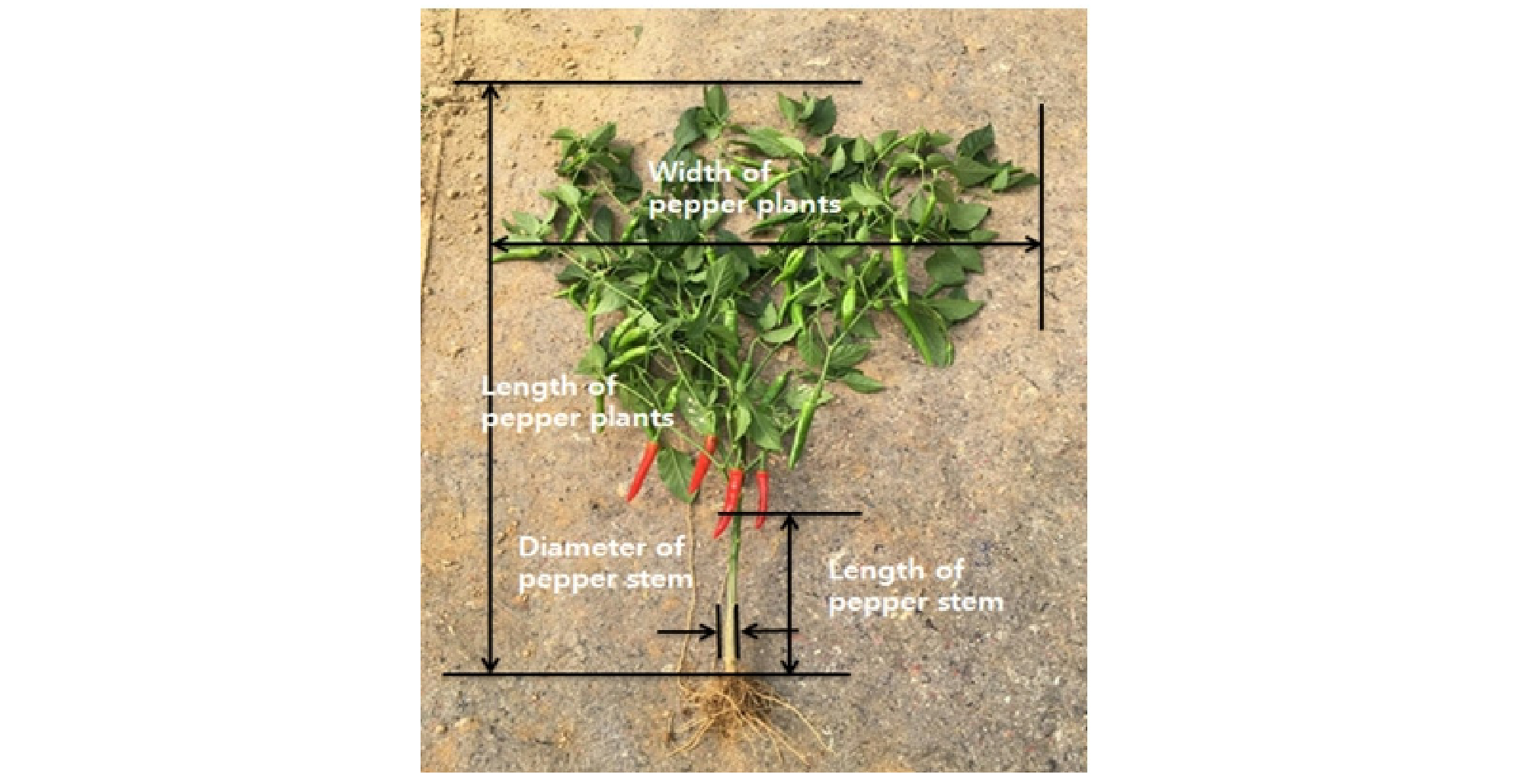##### Fig. 1.

Description of pepper plant dimensions.##### Fig. 2.

Description of pepper fruit dimensions.

The water content in the pepper fruits, pepper stems, and leaves were measured. By using the constant temperature drying method used in Choi (2006), samples were dried at 70°C for 24 h and weighed both before and after drying. As a sample, ten pepper fruit specimens, 50 g of pepper stems, and 50 g of leaves were selected randomly. The measurement results are summarized in Table 3. The average water contents of the pepper fruits, pepper stems, and leaves were 87.61%, 75.60%, and 80.20%, respectively. The water content of pepper fruit was the highest.

Table 3. Moisture content in pepper fruit, stems, and leaves

 Item Weight before drying (g) Weight after drying (g) Moisture content [wet basis (w.b.)] (%) Pepper fruit 120.23 14.9 87.61 Pepper stem 50.00 12.2 75.60 Leaf 50.00 9.9 80.20

Measured properties and definitions

The main properties necessary for particle behavior analysis are Poisson’s ratio, modulus of elasticity, shear modulus, density, coefficient of restitution, and coefficient of friction. Poisson’s ratio, modulus of elasticity, shear modulus, and density are intrinsic properties of each particle, and the coefficients of restitution and friction are properties that are determined through interactions between particles or a particle and a surface. Each of these properties are defined below.

① Modulus of elasticity, shear modulus, and Poisson’s ratio: A slope of the initial straight line in a normal stress–strain curve obtained through a tensile test of a specimen is known as the modulus of elasticity. A compression test, in which a specimen is compressed, can also be performed to obtain the same modulus of elasticity as that in the tensile test. The value can be determined from Hooke’s law, as shown in Equation (1). The upper limit of the normal stress where Hooke’s law applies is called the proportional limit.

$\mathrm{E}=\frac{\mathrm{\sigma }}{\mathrm{\epsilon }}$    (1)

where, E = Modulus of elasticity, MPa

σ = Normal stress within proportional limit, MPa

ε = Normal strain corresponding to the normal stress σ

An initial linear slope on a shear stress–strain curve that is obtained from a torsion test on a specimen is called the shear modulus. The shear modulus can be determined from Hooke’s law on shear stress–strain, as shown in Equation (2).

$\mathrm{G}=\frac{\mathrm{\tau }}{\mathrm{\gamma }}$    (2)

where, G = Shear modulus, MPa

τ = Shear stress, MPa

γ = Shear strain corresponding to the shear stress τ

Poisson’s ratio is defined as the ratio of the lateral strain to the axial strain when a normal stress lower than the proportional limit is applied to the material. This ratio is an important constant for characterizing a material (Beer et al., 2014). The same Poisson’s ratio can be obtained from tension and compression tests, and is defined in Equation (3).

$\mathrm{v}=-\frac{{\mathrm{\epsilon }}_{1}}{{\mathrm{\epsilon }}_{2}}$    (3)

where, ν = Poisson’s ratio

ε1 = Lateral strain

ε2 = Axial strain

The shear modulus can also be calculated using the modulus of elasticity and Poisson’s ratio, as shown in Equation (4) (Beer et al., 2014).

$\mathrm{G}=\frac{E}{2\left(1+\mathrm{v}\right)}$    (4)

② Density: It is defined as the mass per unit volume of a material, as shown in Equation (5).

$\mathrm{\rho }=\frac{m}{V}$    (5)

where, ρ = Density of a material, kg/m3

m = Mass of a material, kg

V = Volume of a material, m3

③ The coefficient of restitution: It indicates the tendency of two objects to bounce after a collision, and is defined as the ratio of the relative velocity immediately after the collision to the relative velocity immediately before the collision (Equation (6)).

$\mathrm{e}=\frac{{\nu }_{1}-{\nu }_{2}}{{\nu }_{1}-{\nu }_{2}}$    (6)

where, e = Coefficient of restitution

u1, u2 = Velocities of particles 1 and 2 just before collision, m/s

v1, v2 = Velocities of particles 1 and 2 just after collision, m/s

In the case of a collision between a particle and a flat surface, because the velocity of the flat surface before and after the collision is zero, the coefficient of restitution can be calculated as shown in Equation (7), where particle 2 is set as the flat surface. Assuming that energy is conserved before and after collision, the coefficient of restitution can be derived as shown in Equation (8), by using the drop height and bounce height.

${e}_{s}=\frac{{v}_{1}}{{\upsilon }_{1}}$    (7)

where, es = Particle–surface coefficient of restitution

${e}_{s}=\sqrt{\frac{h}{H}}$    (8)

where, H = Dropped height of particle, m

h = Bounced height of particle, m

In the case of a collision between particles, if one particle is fixed and the other moves to collide with the fixed particle, the velocity before the collision for the fixed particle is zero. If the fixed particle is set to particle 2, and it is assumed that the energy is conserved before and after the collision, the coefficient of restitution between the particles can be derived as shown in Equation (9) (Montellano et al., 2012).

${e}_{p}=\frac{{\nu }_{1}-{\nu }_{2}}{{\upsilon }_{1}}=\frac{\sqrt{{H}_{2}}-\sqrt{{H}_{1}}}{\sqrt{{H}_{0}}}$    (9)

where, ep = Particle–particle coefficient of restitution

H0 = Dropped height of particle 1, m

H1 = Bounced height of particle 1, m

H2 = Bounced height of particle 2, m

④ The coefficient of friction: It indicates the resistance an object encounters as it slides across a surface, and is defined as the ratio of the friction force to the normal force (Equation (10)). The friction force is a force that interferes with the motion of the object and acts in the opposite direction to the direction that the object is moving.

$\mathrm{\mu }=\frac{F}{N}$    (10)

where, μ = Coefficient of friction

F = Friction force, N

N = Normal force, N

As the force on the stationary object is increased, the object will start to move at some point. The friction force when the stationary object starts to move is called the maximum static friction force. The friction force of the moving object is called the kinetic friction force, which always has a constant value regardless of the size of the contact surface or the speed of the object. The coefficient of friction is defined as the coefficient of static friction when the friction force is at the maximum static friction force. Similarly, the coefficient of friction, where the friction force is the kinetic friction force, is defined as the coefficient of kinetic friction. Because the maximum static friction force is always larger than the kinetic friction force, the static friction coefficient is larger than the kinetic friction coefficient.

The coefficient of friction of an object on a surface can be derived from an inclined plane test (Montellano et al., 2012). The external force acting on the object on the inclined plane is shown in Figure 3 (Beer et al., 2016). As the inclination angle increases, the force pushing the object along the slope (Wsinθ) increases, and the friction force (F) obstructing the motion increases. If the object does not move, the pushing force and the friction force are equal. Using the force equilibrium condition and Equation (10), the static friction coefficient can be determined as shown in Equation (11), using the inclination angle at the moment the object begins to move. Furthermore, when the object continues to move, the magnitude of the kinetic friction is always constant. Therefore, when the force that pushes the object is equal to the magnitude of the kinetic friction, the net force acting on the object becomes zero, making the object move at a constant velocity. The inclination angle can be adjusted to find the inclination angle when the object moves at a constant speed, and the kinetic friction coefficient can be determined by Equation (12) using the force equilibrium condition.##### Fig. 3.

Forces acting on the object lying on the inclined plane.

(11)

where, μs = Coefficient of static friction

Fs = Maximum static friction force, N

W = Weight of the object, N

θs = Inclination angle when the object just begins to move, degree

(12)

where, μk = Coefficient of kinetic friction

Fk = Kinetic friction force, N

θk = Inclination angle when the object moves at constant speed, degree

## Measurement Methods

The pepper fruits and pepper stems are the main particles introduced into the separating part of the self-propelled pepper harvester. Therefore, the properties for the pepper fruits and pepper stems were measured.

① Modulus of elasticity, shear modulus, and Poisson’s ratio: Compression tests were performed using a texture analyzer. A disk of 150 mm diameter was used for the compression tool. The pepper fruits and pepper stems were compressed at a constant speed of 25 mm/min, and the axial and lateral deformations were measured using a high-speed camera. Axial and lateral strains were derived using the measured axial and lateral deformations and the original length (Cakir et al., 2002). The axial strain in this measurement corresponds to the normal strain. The modulus of elasticity was calculated by applying Equation (1) to the initial straight line of normal stress–strain curve, in which the normal stress was calculated by using applied load and contact area.

In addition, Poisson’s ratio was derived from Equation (3) using the axial and lateral strains. Finally, the shear modulus was calculated from Equation (4) using the obtained modulus of elasticity and Poisson’s ratio.

Tables 4 and 5 show the specifications of the texture analyzer and the high-speed camera used in this study. The test equipment is shown in Figure 4.

Table 4. Texture analyzer specifications

 Item Specification Model/Company/Nation TA-XT2i/Stable Micro Systems/UK Maximum force (kg) ±5 Force resolution (g) 0.1 Speed range (mm/min) 6~600 Speed accuracy (%) 0.1 Maximum sampling rate (Hz) 500

Table 5. High-speed camera specifications

 Item Specification Model/Company/Nation NX4-S1/IDT/USA Maximum resolution (pixels) 1024×1024 Maximum FPS at maximum resolution (fps) 2,000 Minimum exposure time (μs) 1 Operating temperature (℃) -40~50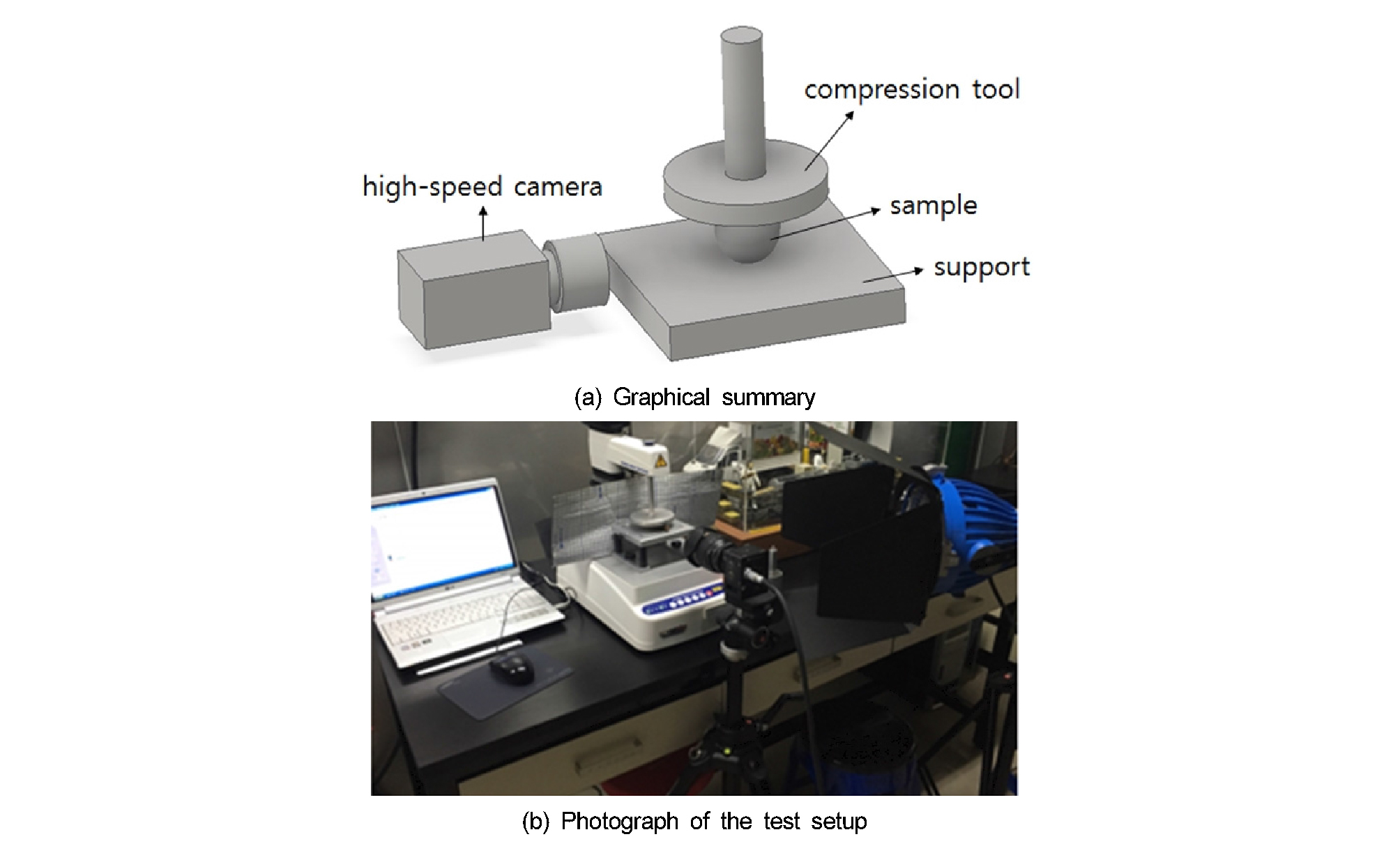##### Fig. 4.

Compression test equipment.

② Density: The densities of pepper fruits and pepper stems were measured using the water pycnometer method (ASTM standards, 2014). A container of known weight was prepared, the surfaces of the materials (i.e., pepper fruits and pepper stems) were coated with a waterproof spray, and the materials were inserted into the container until the container was evenly filled. The filled container was weighed, and the total amount of material was measured. The weight of the empty container was subtracted from the weight of the filled container to calculate the total weight of the materials. The average weight of an individual piece of material was calculated by dividing the total weight of the materials by the total amount of material.

Water was poured into the container to fill the remaining space. By subtracting the volume of the water from the volume of the container, the total volume of material was calculated. By dividing the total volume of the materials by the total amount of material, the average volume of an individual material was obtained.

Then, the density was calculated from the obtained average weight and volume of an individual material using Equation (5) (Figure 5).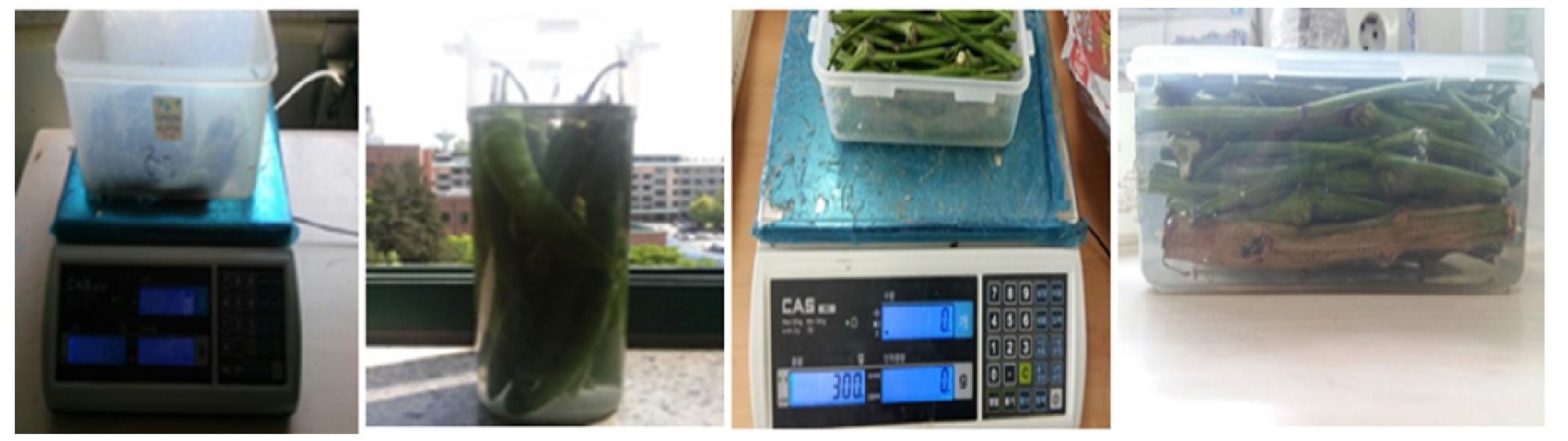##### Fig. 5.

Photograph of density measurements.

③ The coefficient of restitution: The coefficients of restitution between the same materials (pepper fruit–pepper fruit, pepper stem–pepper stem) were obtained using collision tests between particles. Two particles were attached to the end of a thread of the same length, which were then connected to a rod. One particle was suspended vertically and the other particle was moved horizontally at a reference height, H0. The horizontally moving particle was allowed to fall freely and collide with the suspended particle. The bounce height of the two particles after the collision was measured, and the coefficient of restitution was calculated using Equation (9). Figure 6 shows the test method and test image for obtaining the coefficient of restitution between the same materials.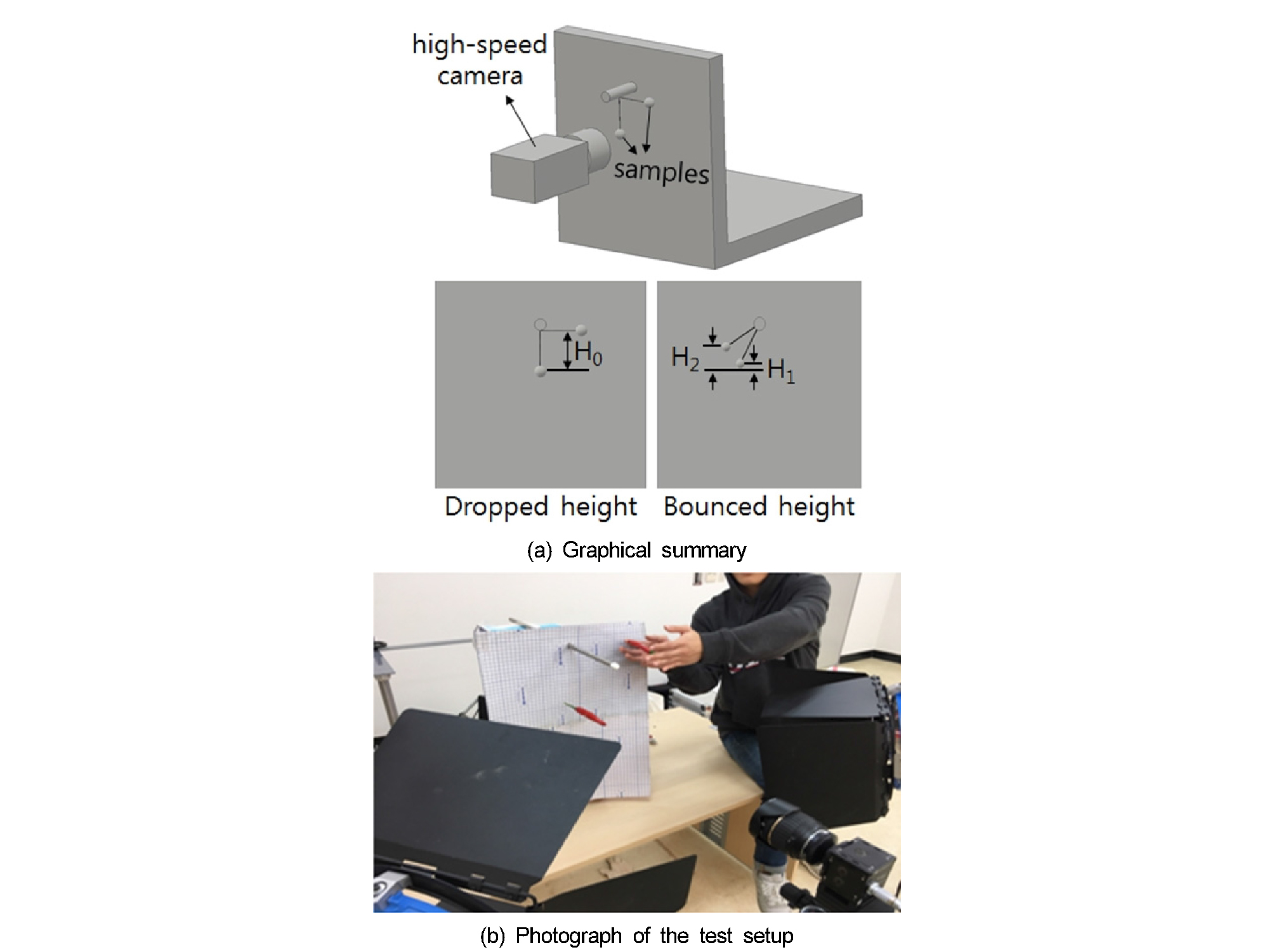##### Fig. 6.

Measurement of particle–particle coefficient of restitution.

The coefficients of restitution between different materials (pepper fruit–pepper stem, pepper fruit–plastic, and pepper stem–plastic) were determined from collision tests between a particle and flat surface. When the coefficients of restitution between a pepper fruit–plastic and a pepper stem–plastic were calculated, a plastic plate was used as the flat surface. When the coefficient of restitution between a pepper fruit and a pepper stem was calculated, pepper stems were used as a flat surface by attaching them to an A4-sized piece of paper without gaps. The particles were held at a height, H, from the flat surface, and fell freely to collide with the flat surface. The bounce height of the particle after the collision was measured, and the coefficient of restitution was calculated using Equation (8). Figure 7 shows the test method and the test image for obtaining the coefficient of restitution between different materials.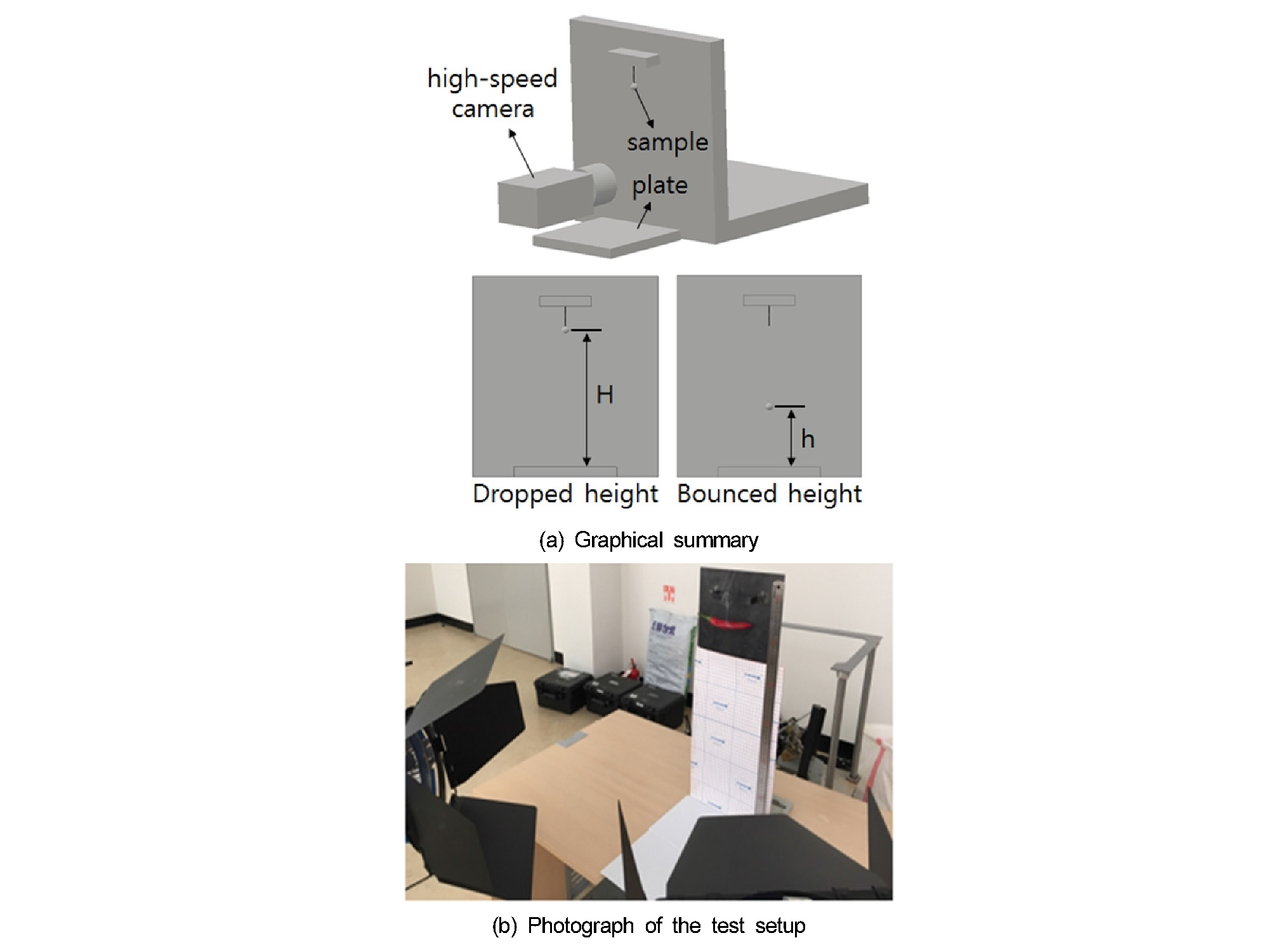##### Fig. 7.

Measurement of particle–surface coefficient of restitution.

The bounce height of the particle was measured using the high-speed camera described in Table 5. To facilitate measurement of the bounce height, a plate with a grid was placed at the back of the test bed. Moreover, the particles were made to collide with each other on their flat parts to prevent the particles from rotating after the collision.

④ The coefficient of friction: The coefficient of friction was calculated using an inclined plane test. The inclination angle was measured using a protractor on the plane, and the movement of the material on the plane was measured using the high-speed camera (Table 5).

The static friction coefficient was obtained by measuring the angle at which the material on the plane started to move (Equation (11)). The coefficients of static friction

between pepper fruit–pepper fruit, pepper fruit–plastic, pepper stem–pepper stem, pepper stem–plastic, and pepper fruit–pepper stem were calculated. At this time, the surface of the other material was closely attached to an A4-sized piece of paper to form an inclined plane. Figure 8 shows the test method and test image for determining the static friction coefficient.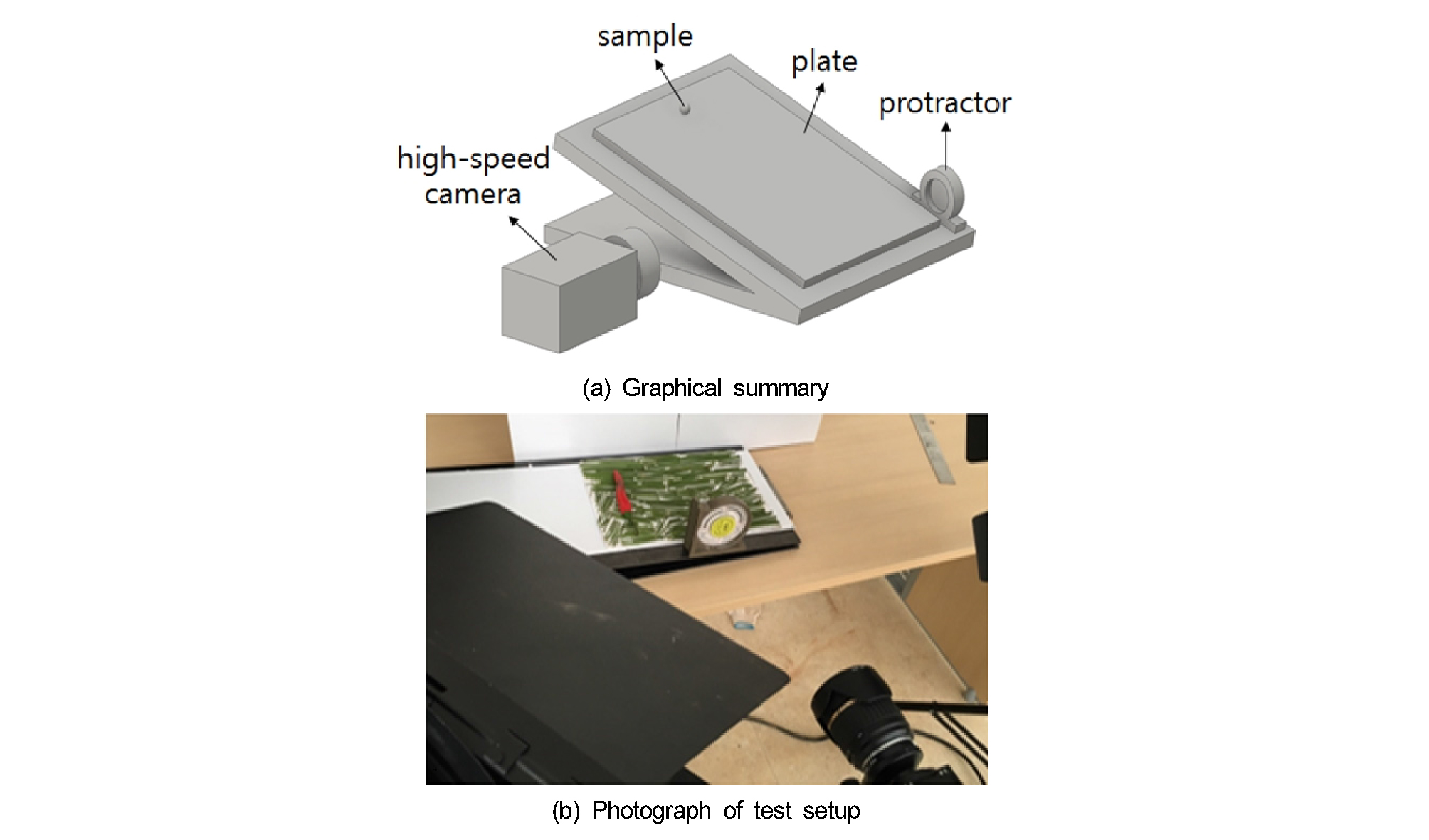##### Fig. 8.

Measurement of coefficient of static friction.

The kinetic friction coefficient was obtained by measuring the angle at which the material on the plane moved at a constant speed (Equation (12)). The kinetic friction coefficients between pepper fruit–plastic and pepper stem–plastic were derived by using a plastic plate as an inclined plane. In order to measure the speed of the material, stripes were drawn on the plate at 50 mm intervals in the longitudinal direction of the plane. The test method and the test image for deriving the kinetic friction coefficients are shown in Figure 9.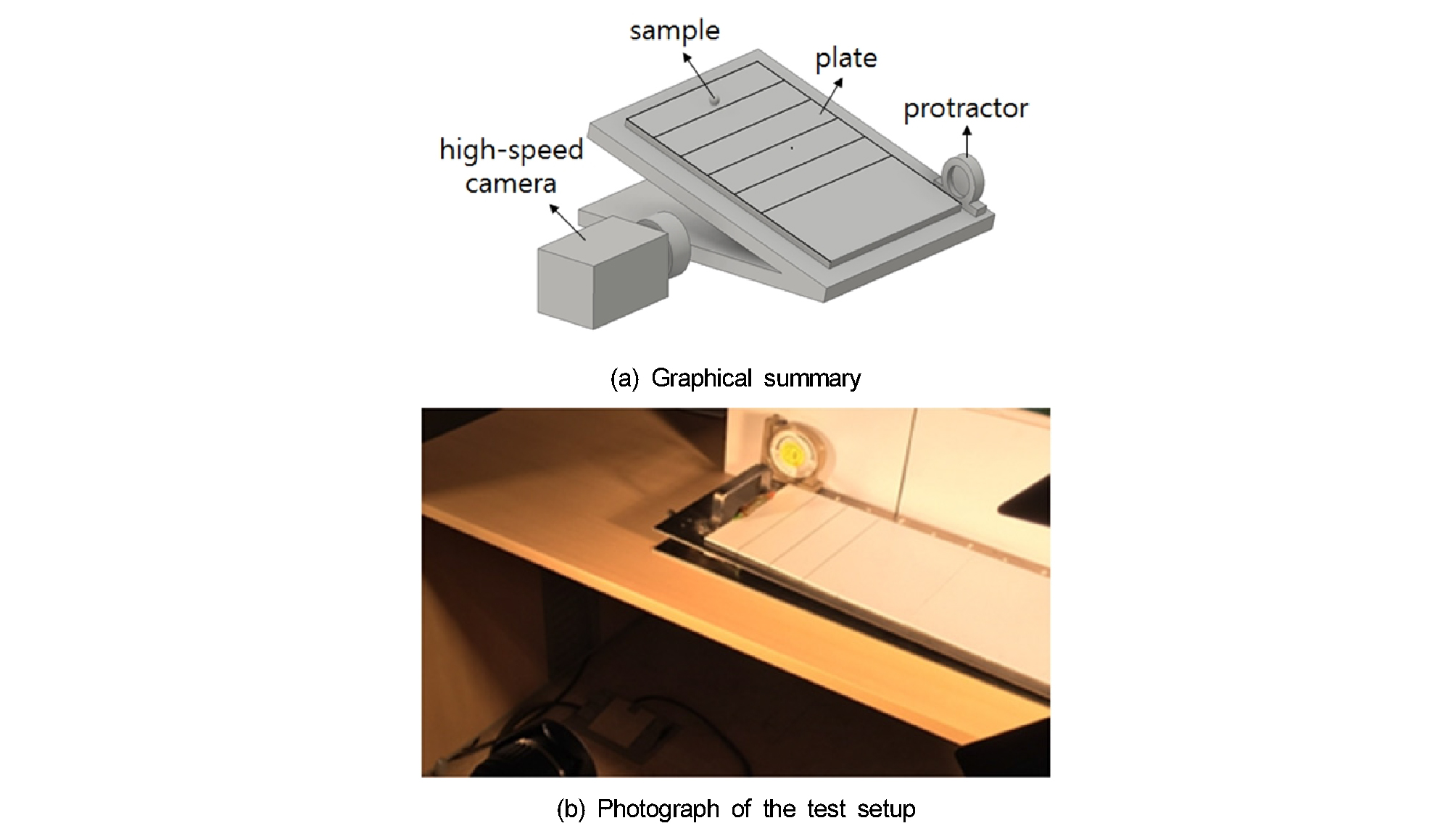##### Fig. 9.

Measurement of coefficient of kinetic friction.

## Results and Discussion

The compression test was repeated five times to obtain Poisson’s ratio, the modulus of elasticity, and the shear modulus. The collision test was repeated three times to obtain the coefficient of restitution, and the incline plane test was repeated three times to obtain the coefficient of friction. The density was derived through a single test using a sufficient number of samples. The average values were used as representative values. In the collision test, the maximum value, the minimum value, and the standard deviation value were also described because the deviation of measurement results could be large depending on the collision surface or the shape of the samples.

① Modulus of elasticity, shear modulus, and Poisson’s ratio: The values of Poisson’s ratio for the pepper fruit and the pepper stem are shown in Table 6, and the modulus of elasticity and shear modulus values are shown in Table 7. Poisson’s ratios for the pepper fruit and pepper stem were not significantly different, but the modulus of elasticity and shear modulus of the pepper stem were higher than those of the pepper fruit by a factor greater than 2.5.

Table 6. Poisson's ratio

 Item Poisson's ratio Pepper fruit 0.295 Pepper stem 0.291

Table 7. Modulus of elasticity and shear modulus

 Item Modulus of elasticity (Pa) Shear modulus (Pa) Pepper fruit 1.152×107 4.624×106 Pepper stem 3.295×107 1.276×107

② Density: Table 8 shows the density measurement results for the pepper fruit and the pepper stem. The density of the pepper stem was more than 1.5 times that of the pepper fruit.

Table 8. Density

 Item Density (kg/m3) Pepper fruit 601.8 Pepper stem 980.4

③ The coefficient of restitution: Table 9 shows the measurement results for the coefficients of restitution between the same materials: pepper fruit–pepper fruit and pepper stem–pepper stem. The coefficient of restitution between pepper fruits was higher than that between pepper stems.

Table 9. Coefficient of restitution between the same materials

 Item Coefficient of restitution Average value Maximum value Minimum value Standard deviation Pepper fruit-Pepper fruit 0.383 0.416 0.336 0.034 Pepper stem-Pepper stem 0.218 0.322 0.135 0.078

Table 10 shows measurements of the coefficients of restitution between the different materials: pepper fruit–plastic, pepper stem–plastic, and pepper fruit–pepper stem. The coefficient of restitution between the pepper fruit and plastic was the greatest, and the coefficient of restitution between pepper stems and plastic was the smallest.

Table 10. Coefficient of restitution between different materials

 Item Coefficient of restitution Average value Maximum value Minimum value Standard deviation Pepper fruit-Plastic 0.399 0.429 0.374 0.023 Pepper stem-Plastic 0.148 0.174 0.122 0.021 Pepper fruit-Pepper stem 0.277 0.299 0.254 0.032

④ The coefficient of friction: Table 11 shows the measured coefficients of static friction. The friction coefficient between pepper fruits was the greatest, and the friction coefficient between pepper fruit and pepper stem was the smallest.

Table 11. Coefficient of static friction

 Item Coefficient of static friction Pepper fruit-Pepper fruit 0.455 Pepper fruit-Plastic 0.364 Pepper stem-Pepper stem 0.332 Pepper stem-Plastic 0.404 Pepper fruit-Pepper stem 0.306

Table 12 shows the kinetic friction coefficient measurements. The coefficient of friction between pepper fruit and plastic was higher than that between pepper stems and plastic.

Table 12. Coefficient of kinetic friction

 Item Coefficient of kinetic friction Pepper fruit-Plastic 0.043 Pepper stem-Plastic 0.034

## Conclusions

In this study, physical and mechanical properties were measured for the domestic pepper variety “AR Legend” for particle behavior analysis. The measured properties were Poisson’s ratio, the modulus of elasticity, shear modulus, density, coefficient of restitution, and coefficient of friction. The measurement methods were determined from a literature review.

Poisson’s ratio, the modulus of elasticity, and the shear modulus were determined from compression tests, and density was determined using the water pycnometer method. Furthermore, the coefficient of restitution was determined from the collision test, and the coefficient of friction was determined from the inclined plane test. Poisson’s ratio, the modulus of elasticity, shear modulus, and density were measured for pepper fruits and pepper stems. The coefficient of restitution and coefficient of friction were derived from contact between various combinations of materials.

By using the properties that were determined in this study, it will be possible to analyze the behavior of pepper fruits and pepper stems during the separating process in a self-propelled pepper harvester. This will allow the performance of the separating part to be analyzed quickly. The accuracy of the measured properties will be verified through a follow-up study.

## Conflict of Interest

No potential conflict of interest relevant to this article was reported.

## Acknowledgements

This work was supported by Korea Institute of Planning and Evaluation for Technology in Food, Agriculture, Forestry and Fisheries (IPET) through Agriculture, Food and Rural Affairs Research Center Support Program, funded by Ministry of Agriculture, Food and Rural Affairs (MAFRA)(716001-7).

## References

1

Alibas, L and N. Koksal. 2015. Determination of physical, mechanical, and structural seed properties of pepper cultivars. International Agrophysics 29: 107-113.https://doi.org/10.1515/intag-2015-0015

10.1515/intag-2015-0015
2

ASTM standards. 2014. D854: Standard test methods for specific gravity of soil solids by water pycnometer. West Conshohocken, PA: ASTM.https://doi.org/10.1520/d0854

10.1520/D0854
3

Barnwal, P., K. K. Singh, A. Sharma, A. K. Chaudhary, T. J. Zachariah and S. N. Saxena. 2015. Selected physical and mechanical properties of black pepper seed (cv. Panniyar-5). International Journal of Seed Spices 5(2): 20-25.

4

Beer, F. P., E. R. Johnston and D. F. Mazurek. 2016. Vector mechanics for engineers: Statics. 11th ed. Ohio, USA: McGraw-Hill Education.

5

Beer, F. P., E. R. Johnston, J. T. Dewolf and D. F. Mazurek. 2014. Mechanics of materials. 6th ed. Ohio, USA: McGraw-Hill Education.

6

Cakir, E., F. Alayunt and K. Ozden. 2002. A study on the determination of Poisson’s ratio and modulus of elasticity of some onion varieties. Asian Journal of Plant Sciences 1(4): 376-378. https://doi.org/10.3923/ajps.2002.376.378

10.3923/ajps.2002.376.378
7

Choi, Y. 2006. Development of the pepper mechanical harvesting system. Unpublished PhD diss. Gwangju, Chonnam: Chonnam National University, Department of Agricultural Engineering. (In Korean, with English abstract)

8

Choi, Y., Y. K. Kim, H. J. Jun, C. H. Lee, J. T. Hong and S. N. Yoo. 2008. Mechanical properties for development of the pepper mechanical harvesting system. The Proceedings of the Korean Society for Agricultural Machinery 13(1): 119-124 (In Korean).

9

Choi, Y., H. J. Jun, C. K. Lee, C. S. Lee, S. N. Yoo, S. R. Suh and Y. S. Choi. 2010. Development of a mechanical harvesting system for red pepper(I) -surveys on conventional pepper cultivation and mechanization of pepper harvesting-. Journal of Biosystems Engineering 35(6): 367-372 (In Korean, with English abstract).https://doi.org/10.5307/jbe.2010.35.6.367

10.5307/JBE.2010.35.6.367
10

Jo, Y. J., M. H. Kim, Y. S. Kang and D. C. Kim. 2016. Development of self-propelled pepper harvester(III) -factorial experiment of separating part-. The Proceedings of the Korean Society for Agricultural Machinery 21(1): 83-84 (In Korean).

11

Jo, Y. J., Y. S. Kang, J. S. Nam, J. S. Choe, E. Inoue, T. Okayasu and D. C. Kim. 2018. Performance Analysis for a Card Cleaner Type Separating System of Pepper Harvester. Journal of the Faculty of Agriculture, Kyushu University 63 (1): 103-111.

12

Kim, Y. B., S. K. Lee, S. T. Kim, W. J. La, D. B. Song and H. J. Lee. 2001. Analysis of Geometrical and Physical Properties of Red Pepper by Machine Vision. Journal of Biosystems Engineering 26(3): 287-294 (In Korean, with English abstract).

13

KOSIS. 2017. 2015 Mechanization Ratio of farm products. Korean Statistical Information Service. Available at: http://kosis.kr. (In Korean)

14

KOSTAT. 2017. 2016 Current state of farm mechanization. STATISTICS KOREA. Available at: www.index.go.kr/ potal/main/EachDtlPageDetail.do?idx_cd=1288. (In Korean)

15

KREI. 2010. Survey on Global consumption trends in Chile pepper products. Research report No. 2010-09. Korea Rural Economic Institute. (In Korean, with English abstract)

16

Montellano, C. G., J. M. Fuentes, E. A. Tellez and F. Ayuga. 2012. Determination of the mechanical properties of maize grains and olives required for use in DEM simulations. Journal of Food Engineering 111: 553-562.https://doi.org/10.1016/j.jfoodeng.2012.03.017

10.1016/j.jfoodeng.2012.03.017
17

Montellano, C. G., A. Ramirez, E. Gallego and F. Ayuga. 2011. Validation and experimental calibration of 3D discrete element models for the simulation of the discharge flow in silos. Chemical Engineering Science 66: 5116-5126.https://doi.org/10.1016/j.ces.2011.07.009

10.1016/j.ces.2011.07.009
18

Nam, J. S., Y. S. Kang, S. B. Kim and D. C. Kim. 2017. Factorial Experiment for Drum-type Secondary Separating Part of Self-propelled Pepper Harvester. Journal of Biosystems Engineering 42(4): 242-250.https://doi.org/10.5307/JBE.2017.42.4.242

19

Park, S. H., K. S. Kang, S. J. Park, Y. S. Kang and D. C. Kim. 2016. Development of self-propelled pepper harvester (II) -gross harvester efficiency depending on cultivar-. The Proceedings of the Korean Society for Agricultural Machinery 21(1): 81-82 (In Korean).

20

Park, S. H., S. J. Park, Y. S. Kang and D. C. Kim. 2015. Development of self-propelled pepper harvester(I) -field test of crop divider-. The Proceedings of the Korean Society for Agricultural Machinery 20(2): 103-104 (In Korean).

21

Rokayya, S. and E. Khojah. 2016. Physical-mechanical estimation of pepper (Capsicum annuum L.) fruit varieties. Journal of Northeast Agricultural University 23(3): 61-69.https://doi.org/10.1016/s1006-8104(16)30060-5

10.1016/S1006-8104(16)30060-5